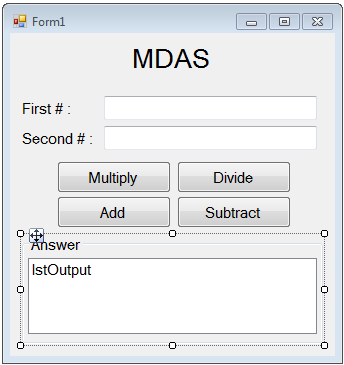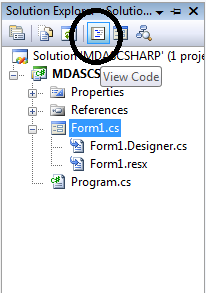# MDAS Calculator In C#

## MDAS Calculator In C#Now, in this tutorial, I will show you how to create MDAS calculator in C#. MDAS are the four fundamental operations in the calculation which stand for Multiplication, Division, Addition and Subtraction that are very common to remember, sounds good right? So, if you want to create your own MDAS calculator? These easy steps below will guide you on how to do it.

## Let’s begin:

Open Microsoft Visual Studio and create a new windows form application. After that, do the form as follows.Go to the solution explorer, hit the “code view” to fire the code editor.In the code editor, declare a variable to represent the total of the MDAS.

` double total = 0;`

Do the following codes for Multiplication methods.

``` private void btnMultiply_Click(object sender, EventArgs e)
{
//set a formula for multiplication
total = double.Parse (txtFinput.Text) * double.Parse (txtSinput.Text);
lstOutput.Items.Add(double.Parse (txtFinput.Text) + " * " + double.Parse (txtSinput.Text) + " = " + total);
}```

Do the following codes for Division methods.

```private void btnDivide_Click(object sender, EventArgs e)
{
//set a formula for Division
total = double.Parse(txtFinput.Text) / double.Parse(txtSinput.Text);
lstOutput.Items.Add(double.Parse(txtFinput.Text) + " / " + double.Parse(txtSinput.Text) + " = " + total);
}```

Do the following codes for Addition methods.

``` private void btnAdd_Click(object sender, EventArgs e)
{
total = double.Parse(txtFinput.Text) + double.Parse(txtSinput.Text);
lstOutput.Items.Add(double.Parse(txtFinput.Text) + " + " + double.Parse(txtSinput.Text) + " = " + total);
}```

Do the following codes for Subtraction methods.

``` private void btnSubtract_Click(object sender, EventArgs e)
{
//set a formula for subtraction
total = double.Parse(txtFinput.Text) - double.Parse(txtSinput.Text);